Project Methodology of the project.

1. Review secondary data

The project for constructing standing tree carbon equations required many supported information. The data of Mae Huad Sector was reviewed by using the old documents derived from the government responsible agencies, namely, the Royal forest department (RFD) and the Department of National Parks, Wildlife and Plant Conservation (DNP). These data consisted of the area of Mae Huad Sector, topography, old forest type area, and land-use change.

These data was used for forest inventory planning to collect tree species list and establish the sample points in the study area in the next step.

2. Field inventory for tree species list

The distribution of sampling points in Mae Huad Sector, Ngao Demonstration forest were determined from a forest inventory using a systematic sampling design with 3 x 3 km fixed grid, with a random start (Figure 1). In each sampling point, using the point sampling technique, tree species were identified and recorded as similar as the record of diameter at breast height (DBH), total height (H) and merchantable height.Figure 1 Map of Mae Huad Sector at NDF illustrating sample point distribution

3. Tree species classification

3.1 Tree collected data from the field inventory was analyzed to obtain basal area (BA), the number of species, wood density in each species, simple statistical description and the Important Value Index (IVI).

3.2 In each forest types, the sample tree species were grouped by using wood density values for 10 groups from the lowest to the highest density classes. The total number of species groups were 30 groups.

3.3 A total of 30 major tree species were selected from each species group with the criteria of the highest IVI.

3.4. The diameter classes of each major tree species were ranged into 3 classes of small, medium and large DBH classes.

4. Sample tree collection and wood sample extraction

4.1 The 30 major tree species in 3 forest types (10 species each forest type) with 3 diameter classes were selected for collecting wood samples, using purposive stratified sampling for 5 sample trees in each diameter class. The total number of the selected trees were 450 trees.

4.2 Tree DBH, total height, merchantable height, and bark thickness were recorded. The upper-stem diameters of each 2-meter sections from the base up to the first major branch were measured by using Wheeler Pentaprism Caliper. The upper stem diameters were used to calculate the tree wet volume (Figure 2).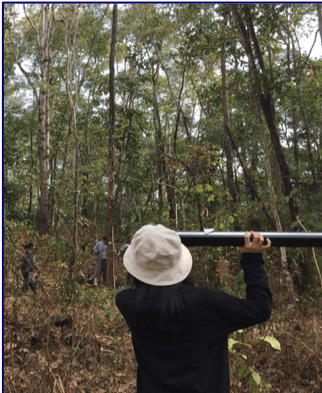Figure 2 The upper stem diameter measurement using Wheeler Pentaprism Caliper

4.3 Wood specimens were collected from the selected trees in the north and the east directions using an increment borer or a chainsaw at DBH (1.3-meter height) (Figure 3). The extracted specimens were suddenly measured the wet volume and wet weight. The total wood specimens were 900 samples from 450 selected trees.Figure 3 Wood specimen collection for carbon content analysis

5. Wood Carbon fraction analysis

In this step, wood samples were analysed by using combustions methods following Kraenzel et al. (2003) and Wutzler et al. (2006). Tree volumes were estimated by using Smalian’s formula or Newton’s formula and wood sample volumes were estimated by using cubic formula. The wood samples were dried in air dry oven at 80 °C for 24 – 48 hours until their weights did not change in order to determine the final dry weights. The 2 dry samples from the same tree were then pulverized together by using a crushing machine to derive a 100-gram dry weight for carbon content analysis. The pulverized sample in each tree were taken for carbon content analysis by using a carbon analyzer (e.g. PerkinElmer 2400 series II CHNS/O Elemental Analyzer). A value of carbon proportion was obtained as a percentage of dry weight and could be converted to the a percentage of wet weight following the studies of Duangsathaporn et al. (2011) and Kantawan (2018). The carbon fraction could be used to estimate the standing tree bole carbon content in the next step. The steps of sample preparation and carbon content analysis were illustrated as below;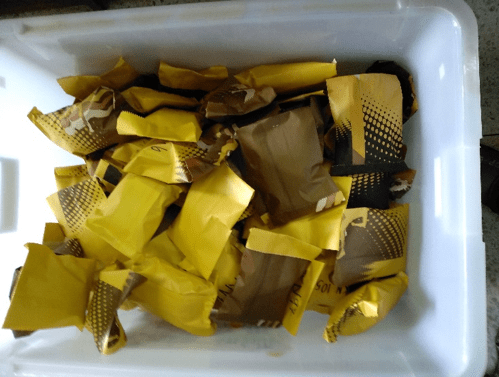Wood samples were dried and weighted to estimate tree carbon contents.Wood samples were pulverized by using a grinder machine.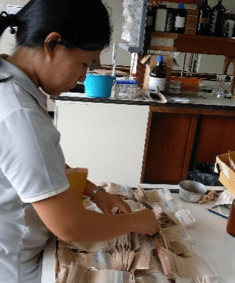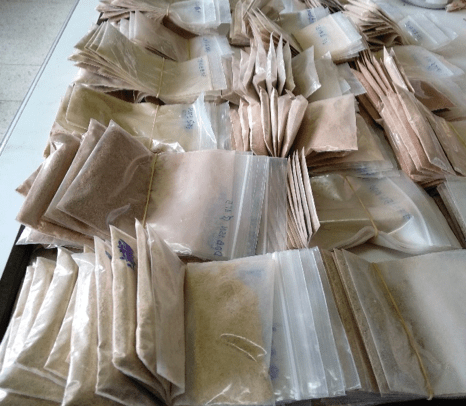Pulverized wood samplesThe specialist analyzed the carbon content of the pulverized wood sample by using the carbon analyzer.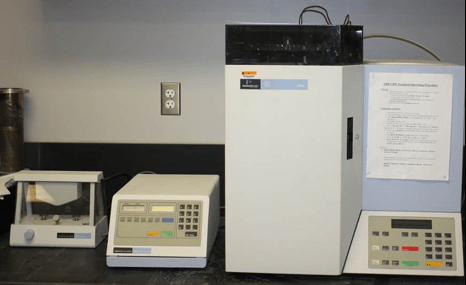The specialist checked the accuracy of carbon contents.The calculation of carbon content in each sample tree by using the new and existing carbon equations.

6. Construct the standing tree carbon equations

The Biometrician developed equations to predict tree bole carbon content from total height and DBH of the standing tree by using the relationship of tree bole carbon content, total height and DBH derived from the collected sample trees and fitting the equations in term of C = ƒ(Total Height, DBH).

7. Estimation of carbon sequestration in each sampling point

The Data Analysts estimated the carbon content in each prism plot by summation of the carbon content in each tree (using the developed new equations) divided by the basal area of itself and multiply with the basal area factor of that point as;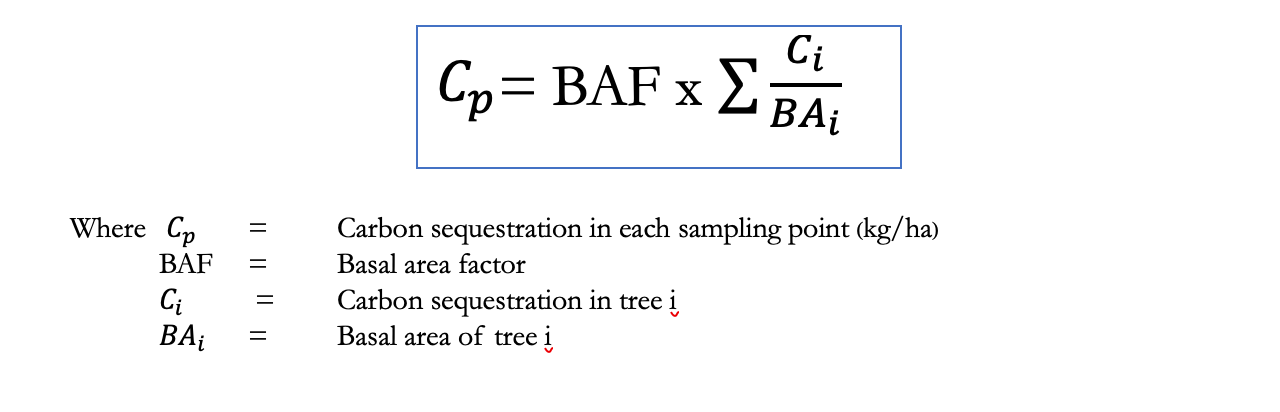8. Construction of the carbon stock map

8.1. Acquire and classify remote sensing data

1) Reviewed background remote sensing data, in particular, reviewed existing satellite data of the project area. The majority satellite data used for this study are from Landsat constellation, the Landsat-8 Operational Land Imager (OLI) and Thermal Infrared Sensor (TIRS), which was a newest satellite in Landsat series.

2) Generated the vegetation index (VI) by means of normalized vegetation index which was a spectral transformation of two image bands (red and near infrared bands) and designed to enhance the contribution of vegetation properties and allowed reliable spatial and temporal inter-comparisons of terrestrial photosynthetic activity and canopy structural variations. This stage attempted to generate Normalized Difference Vegetation Index (NDVI) by utilized  Landsat-8 images that were acquired during the late of 2015, 2016 and 2017, to select the best output for further application i.e., linkage between NDVI and sample carbon plots, on a systematic grid.

8.2 The carbon plots transformation

The carbon plots were transformed from the first inventory plots. Some sample trees were selected and drawn for wood sample for carbon extraction in the Laboratory at Kasetsart University. All trees found in the plots were then converted to mixed stand carbon plots. The total of 54 carbon plots were ready to apply for carbon stock mapping. Adding carbon plots which known coordinate can be done via Excel table through GIS software.

8.3 Carbon plot extraction and regression analysis

The carbon plots became one carbon layer in the GIS database in the point format, when the carbon table with known coordinates were import into the GIS system, while NDVI value represented raster value. In order to create new layer that contain carbon data in the pixel of the raster, those two layers (points shape file and NDVI raster file) were overlaid and merged together, according to that corresponding to the areas defined by the project. The output attribute table insert in the new extract layer contained coordinate points of the carbon plots, NDVI value and carbon details. The best fit and producing highest coefficient of determination derived from the scattering plots between carbon plots and NDVI raster value were selected to generate the carbon stock map.

8.4 Collect and compile ground mapping data

Established new sample plots in the Mae Huad sector of the Ngao Demonstration Forest for carbon stock map accuracy checking. New grids laid out of 3×3 kilometers had been allocated for new sampling points to collect the data of tree species and its dimension; i.e., diameter at breast height and height. Each tree found in the variable sample plots were calculated for carbon sequestration, using the new carbon equations formulated from the first process. The new output of carbon plots is tied with NDVI raster value and repeated the process of regression analysis for choosing the linear model for generating the new carbon pixels according to NDVI raster value. Finally, export the attribute table attached in the new carbon coverage map to summarize the carbon storage in classes.

9. Quality assessment of the carbon map

The new ground-based carbon estimation and those obtained from the carbon stock map at the same corresponding points were then compared. The statistical tests were determined to approve the significant difference between the mean ground and the mean carbon map estimates. The flow diagram depict entire process for carbon stock map generation and classification for Mae Huad sector were shown below in Figure 4.Figure 4 Flow Diagram Depict Entire Process for Carbon Stock Map Generation and Classification for Mae Huad sector, Ngao Demonstration Forest, Lampang Province.